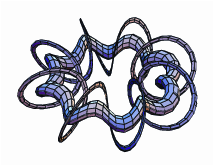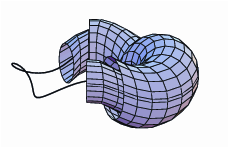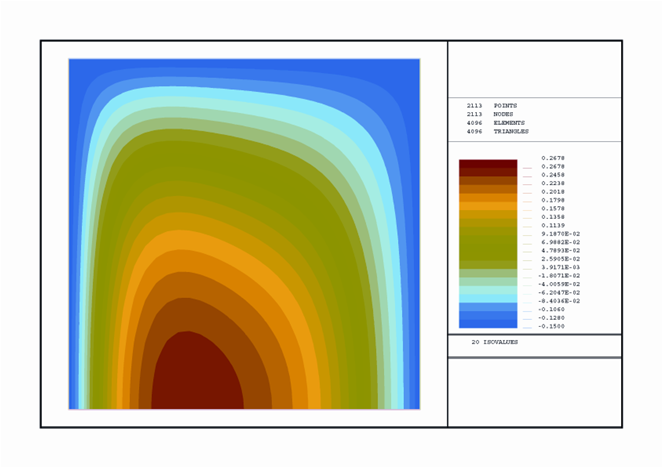## Computer-aided design, computer-aided numerical and engineering simulation (CAD/CAE)

Computer-aided design, computer-aided numerical and engineering simulation (CAD/CAE), also called Numerical Simulation. Numerical simulation integrates mathematical tools that allow modeling, simulating, or predicting the behavior of devices, products and processes in engineering and applied sciences. It emphasizes the numerical simulation of mechanical or structural phenomena, thermal or thermodynamic, acoustic or vibroacoustic, fluid-structure interaction, manufacturing (injection, stamping or forging), electronic or electromagnetic processes, fluids, chemical reactions, phenomena of Combustion, environmental, valuation of financial products and their risks, portfolio management, etc. In this CAD / CAE area, the research groups involved in the Center develop solutions that include all stages, from mathematical modeling to the development of software packages, through mathematical analysis and numerical discretization of the models obtained. As they are transverse technologies, the fields and sectors of application are very diverse; As examples of application of these tools can be mentioned the calculation of the resistance of structures, the design of wind turbines, the solidification of alloys, the heating of induction furnaces, the prediction of the water quality of a lake, the optimization of Combustion in thermal plants, the optimization of the comfort of a vehicle, or the valuation of financial products.

Outline:

• Mathematical modelling and numerical simulation in Engineering and Applied Sciences.• Numerical simulation of coupled problems.
• Mathematical analysis.
• Asymptotic analysis.
• Partial Differential Equations (PDEs): Linear and nonlinear analysis.
• Asymptotic methods. Homogenisation.
• Optimal control. Optimisation.
• Inverse problems.
• Homogenization of periodic materials.
• Differential and functional equations.
• Differential equations.
• Boundary problems for nonlinear differential equations.
• Numerical methods.
• Finite difference methods.
• Finite element methods (FEM).
• Extended finite element methods (XFEM).
• Finite volume methods.
• Boundary Integral Methods (BEM).
• Reduced Order Methods.Specific cases:

• Modelling and simulation in engineering, physics, finance, environmental and applied sciences.
• Solid mechanics:
- Calculation of structures, structures composed of beams, elastic sheets, viscoelastics and viscoplastics.
- Mechanics of contact: contact problems, large deformations, friction, adhesion, fracture and wear.
• Fluid mechanics: hydraulics, gas dynamics.
• Electromagnetism: eddy currents, induction furnaces, electric machines, non-destructive testing.
• Acoustics: sound level, sound insulation, passive and active noise control, absorbent materials.
• Heat transfer: conduction, convection and radiation.
• Chemical reactions: equilibrium, finite kinetics.
• Combustion: thermal power plant boilers.
• Fluid structure interaction: sail and kite design.
• Coupled systems (multiphysics).
• Structural mechanical and acoustic vibrations.
• Biomechanics: numerical simulation and mathematical models of the human jaw and bone formation.
• Mechanical design: steering wheels, orthodontics.
• Environmental Engineering:
- Simulation of the propagation of contaminants, water and air quality.
- Renewable energy: wind, solar, biomass.
• Valuation of financial derivatives.